6

# Authors

The ACES team that worked on the solution is listed in alphabetical order:

Atanas Blagoev ([email protected])

Atanas Panayotov([email protected])

Emil Gyorev ([email protected])

Iliana Voynichka ([email protected])

Slav-Konstantin Ivanov ([email protected])

Ventsislav Yordanov ([email protected])

Even though the news is perceived as one of the most important sources of information to people in current times the information overload problem makes it more and more difficult for users to find news that they are really interested in. Thus, the personalized news recommendation technology has drawn more and more attention from all walks of life. It is the main task in current personalized news recommendation research to design the algorithms with both high recommendation effect and good performance by intelligently combining the existing personalized recommendation technologies with the unique characteristics of news.

As outlined in the current case documentation the main goal is to predict the next best article (not topic) for the visitor of vesti.bg, a Bulgarian news website.

The benefits of having a well-working recommendations engine include (but are not limited to):

• Sustainable increase in the length of stay of the visitors (average session duration)
• Reducing the bounce rate (the percentage of total visitors who abandon the website after visiting just one page)
• Improve customer experience and satisfaction by helping them find what they need more quickly saving them time and effort.

Unlike the recommendation of items in the fields of e-commerce, tourism, movie, music, and so on, the design and application of personalized news recommendation techniques are more complicated and difficult because of the characteristics of news itself. Some of those characteristics are strong contextual correlation, rapid changes of popularity, strong timeliness performance, social impact factors, etc. as well as the relevance between news (news is not independent).

To address the business need ACES has prepared a model that is structured in a way that allows for an hourly refresh. This means that the algorithm is able to automatically ingest the newly created data (articles and click statistics) and generate an updated set of suggested articles that would appear

Last but not least, for the purpose of the challenge, the article that has to be predicted should also be from the provided dataset.

Based on the provided data it is important to state that the current solution does not serve as true recommendation engine but rather a prediction engine that is able to correctly identify the next article read by the customer (without knowing what has been suggested if anything thus we consider it entirely chosen by the reader regardless of other content and suggestions available).

To validate the performance of the model an evaluation dataset will be provided that has the data for the next 1 day (currently unavailable in the dataset). The dataset will include only users and articles already observed in the training dataset.

# Data Understanding

Net Info has provided data with historical visits of articles per user. The data was split in 21 CSV files, each containing 5 columns:

• User ID(anonymized)
• Time – timestamp of when the link was opened by the User
• URL – link to the article on vesti.bg website
• Page Title – the title of the article
• Page Views – number of views accomulated for the period

# Data Preparation

The provided data files were concatenated.

The only way to recognize unique articles is to extract the unique ID that is contained in the URL. Otherwise, two articles with the same name could have different URL extension. By Extracting the ID’s, we were able to create a number of statistics for the data. Furthermore, through the URL we have derived two additional variables: Topic and Subtopic.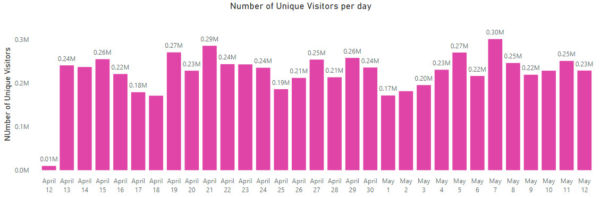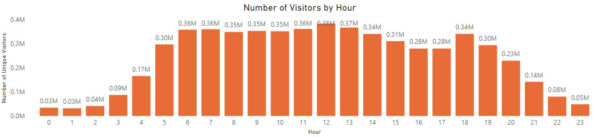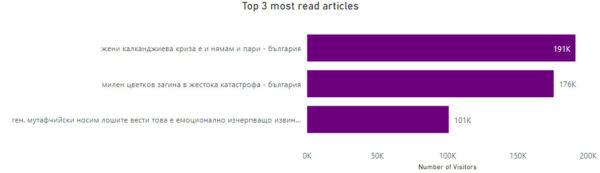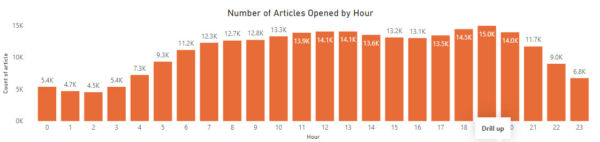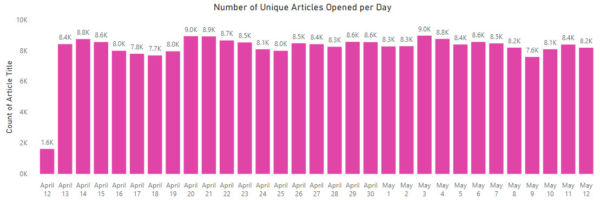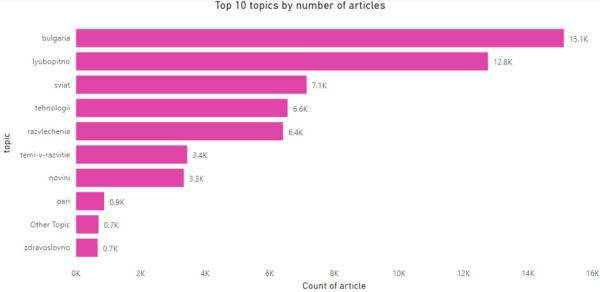Page Title was treated by removing all special symbols (like ! , “, _ etc.)

Unique users were identified by a combination of Page Path and UserID. Then the dataset we de-duplicated by this key.

# Modeling

A Hybrid Deep Learning New Recommender System was used to produce the recommendations. The system can be defined as “hybrid” recommendation system as it relies on both content and collaborative methods to extract information from the data. The architecture is described below:All content based features used within the model are derived prior the training of the neural network. We’ve used 4 groups of such features:

1. Page Topic features – The page topic is extracted from the pagePATH and is then transformed through a one-hot encoder. We’ve used the 10 most common topics for the analysis as they represent almost all of the articles available in the data, as seen in the graphics provided in section “Data Preparation”.
2. Page Title features – The page title is supplied for all articles and can be described as a short description in bulgarian of the article contents. We’ve used a tf-idf transformation to transform the narrative format to a numerical vector. However, the resulting vectors, although sparse, are still very large. To account for that we’ve applied a dimensionality reduction technique by using Truncated SVD to select to top 10 components. This is necessary to speed-up the training and evaluation time.
3. Recency feature – We’ve derived a recency feture, derived as the number of days since the first day the article is seen in the data divided by the number of days available in the data. A simple representation was used due to the limitted time. However given more time we would like to test also the following:
1. Introduce penalties on the loss function related to the age of the article: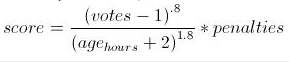2. Introduce “Time-Positional Encodings” inspired by the Transformers framework represented by a numerical vector unique for each number of days since publishing the article. More information is avaialble in the referenced paper:
4. Popularity feature – We’ve derived a popularity feature that represents what proportion of all clicks from the past day are related to the currently analyzed article.

The collaborative filtering algorithm is incorporated within the neural network by applying neural collaborative filtering. This is a procedure that introduces 2 embedding layers – one for the visitors and another one for the articles that are trained by the neural network. The dot product of the 2 encodings is then derived and supplied to the next layers.

The content based features and the output of the neural collborative filtering algorithm are then supplied to a sequence of Dense layers with decreasing number of nodes with final dense layer having only 1 node. Finally, an inception inspired transformation is used that concatenates the output of the final node with the input supplied to the sequence of Dense layers. The concatenated vector is then processed through a sigmoid dense layer with one node to produce the final probability estimate and the log odds used for ranking the articles.

# Evaluation

test_results_2 test_results_1

We’ve uploaded the predicted best articles for all customers in the sample.

# Used Libraries and Technologies

• tensorflow==2.2
• scikit-learn==0.23.0
• numpy==1.18.4
• pandas==1.0.3

# Sources

The chosen architecture is inspired by the following two papers/repos:
– https://github.com/gabrielspmoreira/chameleon_recsys

# CODE

In [ ]:
import pandas as pd
import datetime
import time
import numpy as np
import string
import re


### Data prep process¶

• files were concatinates using shell cat command
• Using pandas, the following operations were performed remove punctuation from pageTitle column remove 'https://' and 'https://' from pagePath column
In [ ]:
#Function to remove unwanted characters from a given string
exclude = ["?", "!", "," , '"', "„", '”', "'", "{", "}", "(", ")", "~", ":", ";"]
def remove_unwanted(x):
try:
x = ''.join(ch for ch in x if ch not in exclude)
x = x.replace("&quot", "")
except:
pass
return x

In [ ]:
#Function to remove 'https://' and 'https://' from a given string
def remove_https(x):
try:
x = ''.join(ch for ch in x if ch not in ['https://', 'https://'])
except:
pass
return x

In [ ]:
#Read in the file
file_name = "/home/datathon/preprocessed_data/vest.csv"

In [ ]:
#Sort by visitor and pageTitle, remove duplicates and keep earliest entry
vest = vest.sort_values(by=['pagePath', 'visitor'])
vest = vest.drop_duplicates(subset=['pagePath', 'visitor'], keep='last')

In [ ]:
#Preprocess data:
# - change title to lowercase
# - remove unwanted characters such as punctuation and &quot
# - remove https:// and https://
vest['pageTitle'] = vest['pageTitle'].str.lower()
vest['pageTitle'] = vest['pageTitle'].apply(remove_unwanted)
vest['pagePath'] = vest['pagePath'].apply(remove_https)
vest['pageTitle'] = vest['pageTitle'].str.strip()
vest['pagePath'] = vest['pagePath'].str.strip()

In [ ]:
#Fix mangled data
#The following operations were performed based on observations
#A lot of the data was removed manually and these steps are not present here
vest.iloc['pagePath'] = 'www.vesti.bg/temi-v-razvitie/tema-koronavirus/zhena-pochina-v-speshna-pomosht-pleven-okaza-se-s-koronavirus-6108950'
vest.iloc['pagePath'] = 'www.vesti.bg/temi-v-razvitie/tema-koronavirus/falshivite-novini-i-covid-19-sega-nakyde--6108600'

#Change rows based on content

In [ ]:
# Create new columns based on the original pagePath. The resulting columns are source, topic, subtopic and article
split_page_path = vest['pagePath'].str.split(pat='/', expand=True)
vest = pd.concat([vest, split_page_path], axis=1)

#Rearange columns
vest.loc[vest.isnull(), [1, 2, 3]] = vest.loc[vest.isnull(), [3, 2, 1]].values
#Rearange columns
vest.loc[vest.isnull(), [1, 2, 3]] = vest.loc[vest.isnull(), [3, 2, 1]].values

vest = vest.replace(np.nan, 'NoTopic', regex=True)

In [ ]:
#Extract id for each article
vest['articleId'] = vest.article.str.extract(pat = '-(\d{7})')

In [ ]:
#Save resulting dataframe
result_file = "/home/datathon/preprocessed_data/vest.csv"
vest.to_csv(result_file, index=False)


In [ ]:
import argparse
import pandas as pd
import numpy as np
import glob
import tensorflow as tf
import json
from sklearn.feature_extraction.text import CountVectorizer, TfidfVectorizer
from sklearn.decomposition import TruncatedSVD

In :
data = pd.read_csv("/home/apanayotov/notebooks/DATATHON/vest.csv",
usecols=["pagePath", "time", "visitor", "topic", "pageTitle"])
data["time"] = pd.to_datetime(data["time"].str[:-4], format="%Y-%m-%d %H:%M:%S")

In :
data.loc[data['topic'].isnull(), "topic"] = "N/A"

In :
data = data[~data['time'].isnull()]

In :
mapping_items = dict(zip(data.pagePath.unique(), range(data.pagePath.unique().shape)))
with open('mapping_items.json', 'w') as fp:
json.dump(mapping_items, fp)

In :
mapping_users = dict(zip(data.visitor.unique(), range(data.visitor.unique().shape)))
with open('mapping_users.json', 'w') as fp:
json.dump(mapping_users, fp)

In :
mapping_topic = dict(zip(data.topic.unique(), range(data.topic.unique().shape)))
with open('mapping_topic.json', 'w') as fp:
json.dump(mapping_topic, fp)

In :
data['pagePath'] = data['pagePath'].map(mapping_items)
data['visitor'] = data['visitor'].map(mapping_users)
data['topic'] = data['topic'].map(mapping_topic)

In :
data['pageTitle'] = data["pageTitle"].str.replace(' \| vesti.bg', '')


In :
keep_topic = data.topic.value_counts().head(10).index.values
data.loc[~data.topic.isin(keep_topic), "topic"] = mapping_topic["N/A"]

In :
NGRAM_RANGE = (1, 2)

In :
tfidf = TfidfVectorizer(ngram_range = NGRAM_RANGE)
data_tfidf = tfidf.fit_transform(data.pageTitle)

In :
svd = TruncatedSVD(n_components = 10)
data_tfidf = svd.fit_transform(data_tfidf)

In :
for idx, col in enumerate([f"tfidf_{x}" for x in range(10)]):
data[col] = data_tfidf[:,idx]

In :
del data_tfidf

In :
data[['pagePath'] + [f"tfidf_{x}" for x in range(10)]].drop_duplicates("pagePath").to_csv("tile_tfidf.csv", index=False)

In :
tile_tfidf = pd.read_csv("tile_tfidf.csv")

In :
data = data.merge(tile_tfidf)

In :
data.drop("pageTitle", axis=1, inplace=True)

In :
data.shape

Out:
(16557333, 14)

# Derive Hot News¶

In :
data['time_yyyyymmdd'] = pd.to_datetime(data.time.dt.date)

In :
hot_news = data.groupby(['time_yyyyymmdd', 'pagePath']).size().reset_index().rename({0:"pageClicks"}, axis=1)

In :
hot_news['popularity_daily'] = hot_news['pageClicks']/hot_news.groupby(['time_yyyyymmdd']).pagePath.transform('count')

In :
hot_news['time_yyyyymmdd'] = hot_news['time_yyyyymmdd'] + pd.Timedelta(1, "D")

In :
hot_news.drop("pageClicks", axis=1, inplace=True)

In :
hot_news.to_csv("hot_news.csv", index=False)


# Derive Min Data And Recency¶

In :
item_opened = data.groupby('pagePath').time_yyyyymmdd.min().reset_index().rename({"time_yyyyymmdd":"min_date"}, axis=1)

In :
item_opened.to_csv("item_opened.csv", index=False)

In :
recent_news = data[['time_yyyyymmdd', 'pagePath']].drop_duplicates().reset_index(drop=True)
recent_news = recent_news.merge(item_opened)

In :
recent_news['recency'] = (recent_news['time_yyyyymmdd'] - recent_news['min_date']).dt.days/32

In :
recent_news.drop("min_date", axis=1, inplace=True)

In :
recent_news.to_csv("recent_news.csv", index=False)

In :
data.shape

Out:
(16557333, 15)

# Store Data¶

In :
hot_news.dtypes

Out:
time_yyyyymmdd      datetime64[ns]
pagePath                     int64
popularity_daily           float64
dtype: object
In :
data.dtypes

Out:
pagePath                   int64
time              datetime64[ns]
visitor                    int64
topic                      int64
tfidf_0                  float64
tfidf_1                  float64
tfidf_2                  float64
tfidf_3                  float64
tfidf_4                  float64
tfidf_5                  float64
tfidf_6                  float64
tfidf_7                  float64
tfidf_8                  float64
tfidf_9                  float64
time_yyyyymmdd    datetime64[ns]
dtype: object
In :
data['time_yyyyymmdd'] = pd.to_datetime(data.time.dt.date)

In :
data = data.merge(hot_news, how="left")

In :
data = data.merge(recent_news, how="left").fillna(0)

In :
data.columns

Out:
Index(['pagePath', 'time', 'visitor', 'topic', 'tfidf_0', 'tfidf_1', 'tfidf_2',
'tfidf_3', 'tfidf_4', 'tfidf_5', 'tfidf_6', 'tfidf_7', 'tfidf_8',
'tfidf_9', 'time_yyyyymmdd', 'popularity_daily', 'recency'],
dtype='object')
In :
dates = data['time_yyyyymmdd'].sort_values().unique()

In :
for idx, date in enumerate(dates):
data[data['time_yyyyymmdd'] == date].drop('time_yyyyymmdd', axis=1).to_csv(f"daily_data/clicks_daily_{idx}.csv", index=False)

In [ ]:



In :
import pandas as pd
import numpy as np
import datetime
import json
import glob


# Prep Data¶

## Extract Data For Last 2 Days¶

In [ ]:
files = glob.glob("/home/apanayotov/notebooks/DATATHON/tmp/daily_data/clicks_daily_*.csv")
data = pd.concat([pd.read_csv(file) for file in files])
data['time'] = pd.to_datetime(pd.to_datetime(data['time']).dt.date)

In :
data.shape

Out:
(16557333, 16)
In :
last_date = data['time'].max()

In :
data = data[data['time'] >= last_date - datetime.timedelta(days=1)]

In :
data.reset_index(drop=True, inplace=True)

In :
keep_topic = data.topic.value_counts().head(10).index.values
data.loc[~data.topic.isin(keep_topic), "topic"] = 0

In :
data = pd.concat([data, pd.get_dummies(data['topic'])], axis=1)

In :
data.columns = ['pagePath', 'time', 'visitor', 'topic', 'tfidf_0', 'tfidf_1',
'tfidf_2', 'tfidf_3', 'tfidf_4', 'tfidf_5', 'tfidf_6', 'tfidf_7',
'tfidf_8', 'tfidf_9', 'popularity_daily', 'recency', 'topic_0',
'topic_1', 'topic_4', 'topic_6', 'topic_7', 'topic_8', 'topic_9',
'topic_12', 'topic_15', 'topic_21', 'topic_34']

In :
data.to_csv("vesti_final_file.csv", index=False)


# Define Keras Model¶

In :
import os
import pandas as pd
import numpy as np
import tensorflow as tf
from tensorflow import keras
import datetime
import swifter
import pickle
import json
from sklearn.utils.extmath import cartesian


In [ ]:
np.random.seed(21)

In :
item_opened = pd.read_csv("tmp/item_opened.csv")

In :
data = pd.read_csv("vesti_final_file.csv").drop(["topic", "time"], axis=1)

In :
data = data[["visitor", "pagePath"] + data.columns[2:].tolist()]

In :
comb = pd.read_csv("vest.csv", usecols=["visitor", "pagePath"]).drop_duplicates()

In :
with open('tmp/mapping_users.json', 'r') as fp:
with open('tmp/mapping_items.json', 'r') as fp:

In :
comb['pagePath'] = comb['pagePath'].map(mapping_items)
comb['visitor'] = comb['visitor'].map(mapping_users)

In :
comb = comb[comb.visitor.isin(data.visitor.unique())]


## Prepare Negative Sample¶

In :
negative_samples = []
for i in range(1):
data_negative = data.copy()
visitors = data_negative["visitor"].values
np.random.shuffle(visitors)
data_negative = data_negative.merge(comb[["visitor", "pagePath"]], how="left", indicator=True)
negative_samples.append(data_negative[data_negative["_merge"] != "both"].drop("_merge", axis=1))

In :
negative_samples = pd.concat(negative_samples).reset_index(drop=True).sample(200000, random_state=21)

In :
mapping_items = dict(zip(data.pagePath.unique(), range(data.pagePath.unique().shape)))
mapping_users = dict(zip(data.visitor.unique(), range(data.visitor.unique().shape)))

data['pagePath'] = data['pagePath'].map(mapping_items)
data['visitor'] = data['visitor'].map(mapping_users)

In :
negative_samples['pagePath'] = negative_samples['pagePath'].map(mapping_items)
negative_samples['visitor'] = negative_samples['visitor'].map(mapping_users)


## Set Up Hyperparameters¶

In :
params = {
"user_column": 0,
"item_column": 1,
"content_features_columns": 2,
"dimensionality": data.shape,
"negative_sample_size": 20,
"embedding_initializer": "glorot_uniform",
"num_users": len(mapping_users),
"num_items": len(mapping_items),
"model_layers": [64, 32, 8, 1],
"mlp_reg_layers": [0.,0.,0.,0.],
"mf_regularization": 0,
"mf_dim": 32,
"softmax_temperature":1,
"batch_size": 2000,
"learning_rate": 0.001,
"beta1": 0.9,
"beta2": 0.999,
"epsilon": 1e-8,
"epochs": 2
}


# Define Model Architecture¶

In :
positive_input = keras.layers.Input(shape=(params["dimensionality"],), name="Positive_Input")

embedding_user = keras.layers.Embedding(
params["num_users"],
params["mf_dim"],
embeddings_initializer=params["embedding_initializer"],
embeddings_regularizer=keras.regularizers.l2(params["mf_regularization"]),
input_length=1,
name="embedding_user")

embedding_item = keras.layers.Embedding(
params["num_items"],
params["mf_dim"],
embeddings_initializer=params["embedding_initializer"],
embeddings_regularizer=keras.regularizers.l2(params["mf_regularization"]),
input_length=1,
name="embedding_item")

model_layers = []
for idx in range(len(params["model_layers"])):
model_layers.append(keras.layers.Dense(
params["model_layers"][idx],
kernel_regularizer=tf.keras.regularizers.l2(params["mlp_reg_layers"][idx]),
activation="relu",
name=f"mlp_{idx}"))

positive_user = keras.layers.Lambda(lambda x: x[:, params["user_column"]])(positive_input)
positive_user_emb = embedding_user(positive_user)

positive_item = keras.layers.Lambda(lambda x: x[:, params["item_column"]])(positive_input)
positive_item_emb = embedding_item(positive_item)

positive_mf_vector = keras.layers.dot([positive_user_emb, positive_item_emb], axes=1)
positive_content_features = keras.layers.Lambda(lambda x: x[:, params["content_features_columns"]:])(positive_input)

positive_sim = tf.keras.layers.concatenate([positive_mf_vector, positive_content_features])

for idx, model_layer in enumerate(model_layers):
if idx == 0:
positive_dense = model_layer(positive_sim)
else:
positive_dense = model_layer(positive_dense)

positive_concat = tf.keras.layers.concatenate([positive_sim, positive_dense])

positive_prob = keras.layers.Dense(1, activation="sigmoid")(positive_concat)

keras_model = tf.keras.Model(
[positive_input],
outputs=positive_prob)

In [ ]:
keras_model.summary()

In :
optimizer = keras.optimizers.Adam(
learning_rate=params["learning_rate"],
beta_1=params["beta1"],
beta_2=params["beta2"],
epsilon=params["epsilon"])

In :
keras_model.compile(optimizer=optimizer, run_eagerly=False, loss="binary_crossentropy")


## Train The Model¶

In :
history = keras_model.fit(
[np.concatenate([data.values, negative_samples.values], axis=0)],
np.concatenate([np.ones(data.shape), np.zeros(negative_samples.shape)], axis=0),
epochs=params["epochs"],
verbose=1)

Epoch 1/2
39935/39935 [==============================] - 3648s 91ms/step - loss: 0.4237
Epoch 2/2
39935/39935 [==============================] - 3741s 94ms/step - loss: 0.1565


## Store The Model¶

In :
keras_model.save("Models/final_model.h5")

In :
keras_model = tf.keras.models.load_model("Models/final_model.h5")

In :
del data, negative_samples


# Evaluate Model¶

In :
# Controls if it's used for evaluation or for final results
test_run = False

In :
import glob
import tqdm
import gc
import os
import pandas as pd
import numpy as np
import tensorflow as tf
from tensorflow import keras
import datetime
import pickle
import json
from sklearn.utils.extmath import cartesian


In :
data = pd.read_csv("vesti_final_file.csv")

In :
with open('tmp/mapping_users.json', 'r') as fp:
with open('tmp/mapping_items.json', 'r') as fp:

In :
params = {
"user_column": 0,
"item_column": 1,
"content_features_columns": 2,
"dimensionality": data.shape,
"negative_sample_size": 20,
"embedding_initializer": "glorot_uniform",
"num_users": len(mapping_users),
"num_items": len(mapping_items),
"model_layers": [64, 32, 8, 1],
"mlp_reg_layers": [0.,0.,0.,0.],
"mf_regularization": 0,
"mf_dim": 32,
"softmax_temperature":1,
"batch_size": 2000,
"learning_rate": 0.001,
"beta1": 0.9,
"beta2": 0.999,
"epsilon": 1e-8,
"epochs": 2
}

In :
mapping_items = dict(zip(data.pagePath.unique(), range(data.pagePath.unique().shape)))
mapping_users = dict(zip(data.visitor.unique(), range(data.visitor.unique().shape)))

data['pagePath'] = data['pagePath'].map(mapping_items)
data['visitor'] = data['visitor'].map(mapping_users)

In :
with open('tmp/mapping_users.json', 'r') as fp:
with open('tmp/mapping_items.json', 'r') as fp:

In :
keras_model = tf.keras.models.load_model("Models/final_model.h5")

In :
data_full = pd.read_csv("vest.csv", usecols=["time", "visitor", "pagePath"])
data_full["time"] = pd.to_datetime(data_full["time"].str[:-4], format="%Y-%m-%d %H:%M:%S").dt.date
data_full["time"] = pd.to_datetime(data_full["time"])
data_full.visitor = data_full.visitor.map(mapping_users_first)
data_full.pagePath = data_full.pagePath.map(mapping_items_first)
data_full.visitor = data_full.visitor.map(mapping_users)
data_full.pagePath = data_full.pagePath.map(mapping_items)
data_full = data_full[(~data_full.pagePath.isnull()) & (~data_full.visitor.isnull())]


## Get All unique Visitors and Pages¶

In :
if test_run:
data_full_visitor = data_full.drop_duplicates(['visitor', 'flag'])
users = data_full_visitor[data_full_visitor.duplicated('visitor')].visitor.unique()
else:
users = data_full.visitor.unique()

In :
if test_run:
items = data_full[data_full['time'] != data_full['time'].max()].pagePath.unique()
else:
items = data_full.pagePath.unique()


## Get context features¶

In :
tfidf_table = data[['pagePath'] + [f"tfidf_{x}" for x in range(10)] + [x for x in data.columns if "topic" in x]].drop_duplicates("pagePath")

In :
hot_news = pd.read_csv("tmp/hot_news.csv")

In :
item_opened = pd.read_csv("tmp/item_opened.csv")
item_opened['min_date'] = pd.to_datetime(item_opened['min_date'])


## Get Exclusion Dataset¶

In :
data = pd.read_csv("vest.csv", usecols=["time", "visitor","pagePath"])
data["time"] = pd.to_datetime(pd.to_datetime(data["time"].str[:-4], format="%Y-%m-%d %H:%M:%S").dt.date)
if test_run:
data = data[data["time"] != data["time"].max()]
data.drop_duplicates(["visitor", "pagePath"], inplace=True)

In :
data.visitor = data.visitor.map(mapping_users_first)
data.pagePath = data.pagePath.map(mapping_items_first)
data['pagePath'] = data['pagePath'].map(mapping_items)
data['visitor'] = data['visitor'].map(mapping_users)
data = data[(~data.pagePath.isnull()) & (~data.visitor.isnull())]

In :
del data_full


## Produce Final Results¶

In [ ]:
results = []
for user_ids_temp in tqdm.tqdm(np.array_split(users, 110)):
X_positive = pd.DataFrame(cartesian((user_ids_temp.tolist(), items.tolist())), columns=["visitor", "pagePath"])
if test_run:
X_positive['time_yyyyymmdd'] = "2020-05-12"
else:
X_positive['time_yyyyymmdd'] = "2020-05-13"
X_positive = X_positive.merge(hot_news, how="left")
X_positive.loc[X_positive['popularity_daily'].isnull(), "popularity_daily"] = 0.
before = X_positive.shape
X_positive = X_positive.merge(item_opened)
if before != X_positive.shape:
raise Exception("Missmatch in data")
X_positive['time_yyyyymmdd'] = pd.to_datetime(X_positive['time_yyyyymmdd'])
X_positive['recency'] = (X_positive['time_yyyyymmdd'] - X_positive['min_date']).dt.days/32
X_positive.drop(["time_yyyyymmdd", "min_date"], axis=1, inplace=True)

before = X_positive.shape
X_positive = X_positive.merge(tfidf_table)
if before != X_positive.shape:
raise Exception("Missmatch in data")
columns = ['visitor', 'pagePath', 'tfidf_0', 'tfidf_1', 'tfidf_2', 'tfidf_3',
'tfidf_4', 'tfidf_5', 'tfidf_6', 'tfidf_7', 'tfidf_8', 'tfidf_9',
'popularity_daily', 'recency', 'topic_0', 'topic_1', 'topic_4',
'topic_6', 'topic_7', 'topic_8', 'topic_9', 'topic_12', 'topic_15',
'topic_21', 'topic_34']
preds = keras_model.predict(X_positive[columns].values, batch_size = 10000, verbose=1)

X_positive['preds'] = preds
data_tmp = data[data['visitor'].isin(user_ids_temp)][['visitor', 'pagePath']].drop_duplicates()
X_positive = X_positive[["visitor", "pagePath", "preds"]].merge(data_tmp, indicator=True, how="left")
result = X_positive[X_positive._merge == "left_only"].sort_values(["preds", "pagePath"], ascending=[False, False]).drop_duplicates("visitor")

del X_positive, data_tmp, preds

#results.append(result[["visitor", "pagePath", "preds"]])
if os.path.exists("results_fin.csv"):
else:
result.to_csv("results_fin.csv", index=False)


## Produce Accuracy Estimate¶

In [ ]:
if test_run:
files = glob.glob("/home/apanayotov/notebooks/DATATHON/tmp/daily_data/clicks_daily_*.csv")
data = pd.concat([pd.read_csv(file) for file in files])
data['time'] = pd.to_datetime(pd.to_datetime(data['time']).dt.date)

data.visitor = data.visitor.map(mapping_users_first)
data.pagePath = data.pagePath.map(mapping_items_first)
data['pagePath'] = data['pagePath'].map(mapping_items)
data['visitor'] = data['visitor'].map(mapping_users)
data = data[(~data.pagePath.isnull()) & (~data.visitor.isnull())]
data = data[(data.pagePath.isin(items)) & (data.visitor.isnull(users))]

data = data[data['time'] == data['time'].max()]
evaluation = data.merge(results[["visitor", "pagePath"]], how="inner", on="visitor")

accuracy = (evaluation['pagePath_x'] == evaluation['pagePath_y']).sum()/data.visitor.unique().shape

In [ ]:



#### 7 thoughts on “ACES solution to article recommender engine case – provided by NetInfo”

1.liad says:
0

Hello, very nice work and nice video too.

1. It seems that the evaluation results are missing – I can see the code, but not the actual output. How well did your approach do, based on the historical data?
2. I’m a bit concerned about the negative sampling approach – couldn’t it also penalize possible links between users and articles?
3. Given that this model goes into ‘production’ and now your client asks you to improve the model accuracy even further, and bring more clicks. Which directions would you take to improve it?

1.strabron says:
0

Hi, Thank you for the feedback!
1. The accuracy estimated for 12th May is 39%. You can find the final results for 13th May here – https://drive.google.com/open?id=1pf00CVy8nqKqpziGZioJTDc-rxm_ahlW . I can also upload the results for 12th of May if you believe that they will be useful.
2. I agree. It could penalize non-observed, but possible future links learned through the collaborative filtering algorithm. I’m not worried about the impact on content based features as in their case the flag is “truly” negative. However, about the collaborativ filtering algorithm, to mitigate this issue we’ve used short embeddings for the embedding layers, to generalize as best as possible the behavior. We believe that this generalization of behavior on visitor + article level will mitigate any negative effect on the final ranking – e.g. the probability estimate will be biased by the negative sampling, but the ranking of the articles (which is most important) given appropraite embedding length will not be.
3. Currently we don’t use the most recently read articles for context, while the literature shows that the most recently read articles are a source of sigificant information about the most likely “next best article” to click. This information is traditionally incorporated through markov chain relationship or through recursive neural networks. However, what I want to test is to supply the last 10-20 context articles and 10-20 negative sampling articles and assess them through a softmax function processed on customer level. This is similar to the approach described in the “chameleon” repositori referenced in our Article. Another possible improvement could come from the “recency” features that we use and the “hot nws” features. We’ve used very basic representation while for “recency” we can use some of the approaches proposed by us above and for “hot news” we can implement features that are dependent on a longer history.

2.strabron says:
0

Hi All,
We noticed that the attached files in the article are not the correct one. They are only 2, while finally we have 25 files with ~2 MB size. We don’t want to change the article as it will move it back to “DRAFT”, so please refer to the results here: https://drive.google.com/open?id=1pf00CVy8nqKqpziGZioJTDc-rxm_ahlW when evaluating the model.

The accuracy of the model on 12th May is 39%. However, we also used this day for training, so the estiamte might be biased.

The accuracy is estiamted following the approach discussed in the DSS chat for the case:
1. First we count the total number of users experiencing article interaction with the user-specific “next best article” proposed by the recommendation system (only one article is allowed per user). E.g. – if a user has clicked on 6 article in the next day we should predict only one of them to count this as “1” regardless of their order in that day.
2. Then we count the number of visitors present in both train and test that have interacted with an article that is present in the “train” set. E.g. – If a user has clicked on 1 article the next day, but this article was not present in the “train” dataset we ignore it. However, if an user clicks on 25 articles and 20 of them were present in the “train” set then we count this as “1”.

Finally we use “1.” as numerator and “2.” as denominator to derive the evaluation metric:
eval_metic = “Count of 1.”/”Count of 2.”

3.metodinikolov says:
0

Nice work and nice video.
A few things I am curious about:
* Have you looked at how the two parts of your algorithm behave on their own: I.e. if two titles are deemed close by the algorithm – are they really so to a human?
* In the same vein to liad’s third question above – say you had the actual articles – how much of a change to your algorithm would this entail?

Best,

1.strabron says:
0

Hi, Thank you for the feedback!

1) If you are refering to the tf-idf + truncated svd representation of the titles. We checked several cases and indeed similar vectors (by cosine similarity) are simialar for a human. As for testing the algorithm using only “Content Based Features” or only “Collaborative filtering”. We didn’t have time to check this, but some preliminary tests using only Neural Collaborative Filtering weren’t as good, so my intuition is that the main added value is currently comming from the “Content Based Features” and the Neural Network transformations afterwards + Negative Sampling

2) I would refrain from introducing the text of the articles for the time being, as in my mind other sources of data as the N most recent articles or the recency and hot news definitions would result in much higher uplift. Our working assumption is that the Title of the article is sufficient summary of the article contents, but we haven’t done any alaysis to validate that.

4.alex-efremov says:
0
1.strabron says: